# Electric Field – Definition, Formula, Electric Field Direction, SI Unit

0
171

## What is Electric Field?

An electric field is said to exist at a point if a force of electrical origin is exerted on a stationary charged body placed at that point. Quantitatively, the electric field or electric intensity or the electric field strength at a point is defined as the force experienced by a unit positive test charge placed at that point, without disturbing the position of source charge.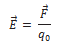The electric field at a point is defined as the electrostatic force per unit test charge acting on a vanishingly small positive test charge placed at that point. Hence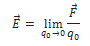The electric field is a vector quantity whose direction is same as that of the force exerted on a positive test charge.

SI unit is Newton per coulomb (NC-1). It is equivalent to volt per meter (Vm-1).

Dimension of Electric field is [MLT-3A-1].

## Electric Field Due to a Point Charge

A single point charge has the simplest electric field.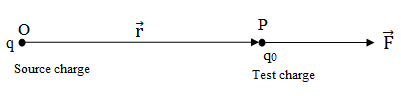According to Coulomb’s law, the force on charge q0 is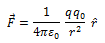Electric field at point P is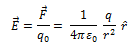The magnitude of the field isClearly, E ∝ 1/r2. This means that at all points on the spherical surface drawn around the point charge, the magnitude of is same and does not depend on the direction of . such a field is called spherically symmetric or radial field, i.e., a field which looks the same in all direction when seen from the point charge.

## Electric Field due to a System of Charges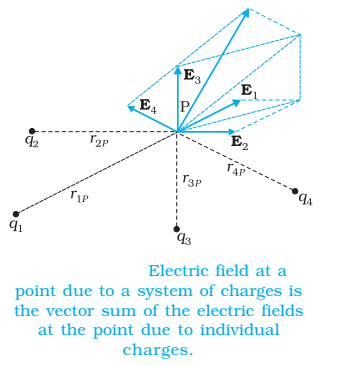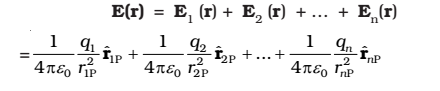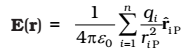is a vector quantity that varies from one point to another point in space and is determined from the positions of the source charges.

### Properties of an Electric Field

1. Field lines never intersect each other.
2. They are perpendicular to the surface charge.
3. The field is strong when the lines are close together, and it is weak when the field lines move apart from each other.
4. The number of field lines is directly proportional to the magnitude of the charge.
5. The electric field line starts from the positive charge and ends from negative charge.
6. If the charge is single, then they start or end at infinity.
7. The line curves are continuous in a charge-free region.